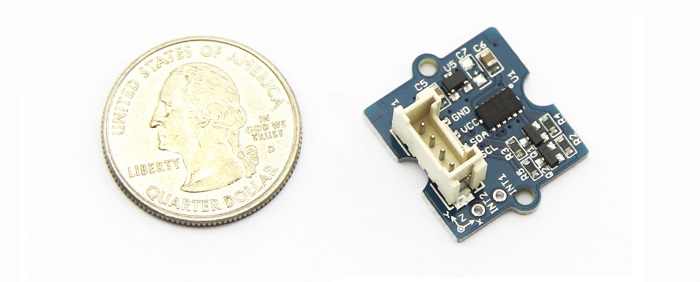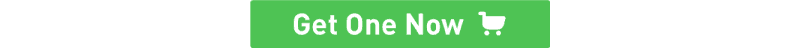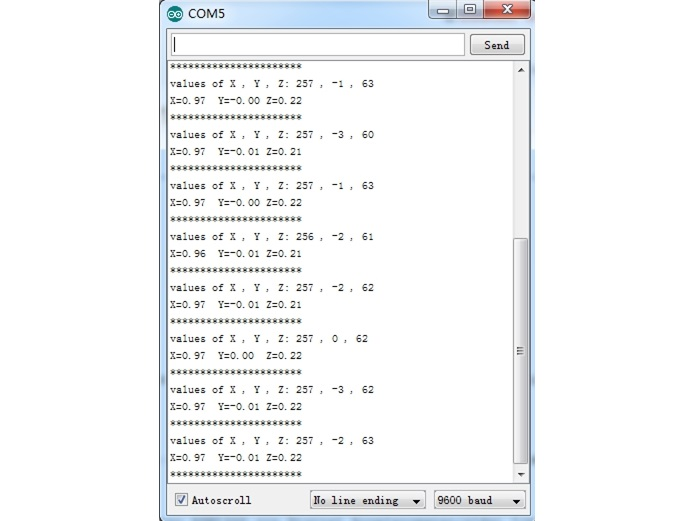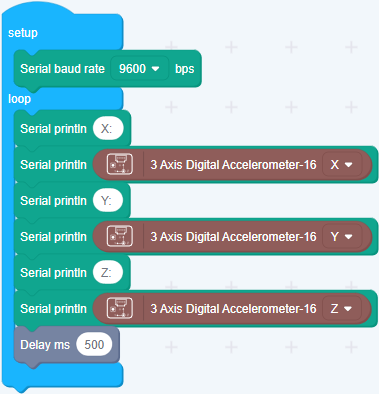edit

# Grove - 3 Axis Digital Accelerometer(±16g)3-Axis Digital Accelerometer is the key part in projects like orientation detection, gesture detection and Motion detection. This 3-Axis Digital Accelerometer(±16g) is based on low power consumption IC ADXL345. It features up to 10,000g high shock survivability and configurable Samples per Second rate. For generous applications that don't require too large measurement range, this is a great choice because it's durable, energy saving and cost-efficient.## Specifications¶

• Working voltage: 3.0 - 5.5V
• Test Range: ±16
• Sensitivity: 3.9mg / LSB
• Standby Current: 0.1μA（Under stand mode Vcc = 2.5 V (typical)）
• 10000 g high shock survivability
• ECOPACK®RoHS and “Green” compliant
• Suli-compatible Library

Tip

## Demonstration¶

With Arduino

Every accelerometer has been individually tested before shipping to you. But in rare cases, you might need to reset the zero-offset by yourself. Here below we show you how to read the raw data and obtain data in the unit of g, AKA g-force, from this accelerometer.• Step1: Plug it onto the I2C port of your Grove - Base Shield.
• Step2: Download the Digital Accelerometer(±16g) Library .zip and unpack it into arduino-1.0\libraries in your Arduino installation folder. If you don't know how to install library for Arduino, please follow the toturial How to install an Arduino library
• Step3： If you have the library installed, Open the demo code directly by the path: ** File(文件) -> Example(示例) ->DigitalAccelerometer_ADXL345->ADXL345_demo_code. **
• Step4： Upload the code and open the serial monitor(usually it is on the right up corner). Please refer to the toturial Upload code if you do not know how to upload.
• Step5： The result will be showed as the format in below image, shake the grove and you will find the number changing.The outputs of this sensor consist of two parts: raw data and 3-axis acceleration info converted into the unit of gravity, "g".

### Play with Codecraft¶

#### Hardware¶

Step 1. Using a Grove cable connect Grove - 3-Axis Digital Accelerometer(±16g) to Seeeduino's I2C port. If you are using Arduino, please take advantage of a Base Shield.

#### Software¶

Step 1. Open Codecraft, add Arduino support, and drag a main procedure to working area.

NoteSuccess

When the code finishes uploaded, you will see the acceleration displayed in the Serial Monitor.

With Raspberry Pi

• Step1: You should have got a raspberry pi and a grovepi or grovepi+.

• Step2: You should have completed configuring the development enviroment, otherwise follow here.• Step3: Connection
• Plug the sensor to grovepi socket i2c-x(1~3) by using a grove cable.

• Step4: Navigate to the demos' directory:

cd yourpath/GrovePi/Software/Python/

To see the code

  nano grovepi_tilt_switch.py   # "Ctrl+x" to exit #

import smbus
from time import sleep

# select the correct i2c bus for this revision of Raspberry Pi
revision = ([l[12:-1] for l in open('/proc/cpuinfo','r').readlines() if l[:8]=="Revision"]+['0000'])
bus = smbus.SMBus(1 if int(revision, 16) >= 4 else 0)

EARTH_GRAVITY_MS2   = 9.80665
SCALE_MULTIPLIER    = 0.004

DATA_FORMAT         = 0x31
BW_RATE             = 0x2C
POWER_CTL           = 0x2D

BW_RATE_1600HZ      = 0x0F
BW_RATE_800HZ       = 0x0E
BW_RATE_400HZ       = 0x0D
BW_RATE_200HZ       = 0x0C
BW_RATE_100HZ       = 0x0B
BW_RATE_50HZ        = 0x0A
BW_RATE_25HZ        = 0x09

RANGE_2G            = 0x00
RANGE_4G            = 0x01
RANGE_8G            = 0x02
RANGE_16G           = 0x03

MEASURE             = 0x08
AXES_DATA           = 0x32

self.setBandwidthRate(BW_RATE_100HZ)
self.setRange(RANGE_2G)
self.enableMeasurement()

def enableMeasurement(self):

def setBandwidthRate(self, rate_flag):

# set the measurement range for 10-bit readings
def setRange(self, range_flag):

value &= ~0x0F;
value |= range_flag;
value |= 0x08;

# returns the current reading from the sensor for each axis
#
# parameter gforce:
#    False (default): result is returned in m/s^2
#    True           : result is returned in gs
def getAxes(self, gforce = False):

x = bytes | (bytes << 8)
if(x & (1 << 16 - 1)):
x = x - (1<<16)

y = bytes | (bytes << 8)
if(y & (1 << 16 - 1)):
y = y - (1<<16)

z = bytes | (bytes << 8)
if(z & (1 << 16 - 1)):
z = z - (1<<16)

x = x * SCALE_MULTIPLIER
y = y * SCALE_MULTIPLIER
z = z * SCALE_MULTIPLIER

if gforce == False:
x = x * EARTH_GRAVITY_MS2
y = y * EARTH_GRAVITY_MS2
z = z * EARTH_GRAVITY_MS2

x = round(x, 4)
y = round(y, 4)
z = round(z, 4)

return {"x": x, "y": y, "z": z}

if __name__ == "__main__":
# if run directly we'll just create an instance of the class and output

print "   x = %.3fG" % ( axes['x'] )
print "   y = %.3fG" % ( axes['y'] )
print "   z = %.3fG" % ( axes['z'] )


5.Run the demo.

    sudo python grove_tilt_switch.py


With Beaglebone Green

To begin editing programs that live on BBG, you can use the Cloud9 IDE. As a simple exercise to become familiar with Cloud9 IDE, creating a simple application to blink one of the 4 user programmable LEDs on the BeagleBone is a good start.

• Step1: Set the Grove - UART socket as a Grove - GPIO Socket, just follow this link.

• Step2: Click the "+" in the top-right to create a new file.

• Step3: Copy and paste the following code into the new tab.

import smbus
import time

bus = smbus.SMBus(1)

EARTH_GRAVITY_MS2   = 9.80665
SCALE_MULTIPLIER    = 0.004

DATA_FORMAT         = 0x31
BW_RATE             = 0x2C
POWER_CTL           = 0x2D

BW_RATE_1600HZ      = 0x0F
BW_RATE_800HZ       = 0x0E
BW_RATE_400HZ       = 0x0D
BW_RATE_200HZ       = 0x0C
BW_RATE_100HZ       = 0x0B
BW_RATE_50HZ        = 0x0A
BW_RATE_25HZ        = 0x09

RANGE_2G            = 0x00
RANGE_4G            = 0x01
RANGE_8G            = 0x02
RANGE_16G           = 0x03

MEASURE             = 0x08
AXES_DATA           = 0x32

self.setBandwidthRate(BW_RATE_100HZ)
self.setRange(RANGE_2G)
self.enableMeasurement()

def enableMeasurement(self):

def setBandwidthRate(self, rate_flag):

# set the measurement range for 10-bit readings
def setRange(self, range_flag):

value &= ~0x0F;
value |= range_flag;
value |= 0x08;

# returns the current reading from the sensor for each axis
#
# parameter gforce:
#    False (default): result is returned in m/s^2
#    True           : result is returned in gs
def getAxes(self, gforce = False):

x = bytes | (bytes << 8)
if(x & (1 << 16 - 1)):
x = x - (1<<16)

y = bytes | (bytes << 8)
if(y & (1 << 16 - 1)):
y = y - (1<<16)

z = bytes | (bytes << 8)
if(z & (1 << 16 - 1)):
z = z - (1<<16)

x = x * SCALE_MULTIPLIER
y = y * SCALE_MULTIPLIER
z = z * SCALE_MULTIPLIER

if gforce == False:
x = x * EARTH_GRAVITY_MS2
y = y * EARTH_GRAVITY_MS2
z = z * EARTH_GRAVITY_MS2

x = round(x, 4)
y = round(y, 4)
z = round(z, 4)

return {"x": x, "y": y, "z": z}

if __name__ == "__main__":
# if run directly we'll just create an instance of the class and output

while True:
print "   x = %.3fG" % ( axes['x'] )
print "   y = %.3fG" % ( axes['y'] )
print "   z = %.3fG" % ( axes['z'] )
time.sleep(2)

• Step4: Save the file by clicking the disk icon with with the .py extension..

• Step5: Connect Grove - 3-Axis Digital Accelerometer(±16g) to Grove I2C socket on BBG.

• Step6: Run the code. You'll sfind that the terminal outputs Gravity info every 2 seconds.

## Projects¶

Grove - Introduction in 3-Axis Digital Accelerometer: How to use a 3-axis digital accelerometer.

## Tech Support¶

Please submit any technical issue into our forum.